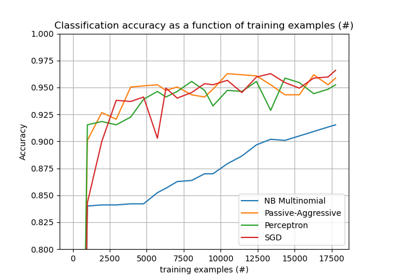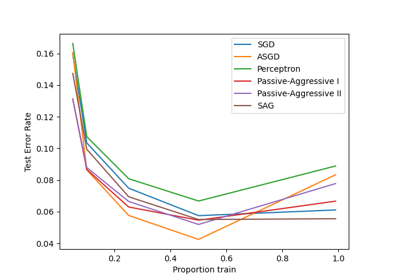# sklearn.linear_model.Perceptron¶

class sklearn.linear_model.Perceptron(*, penalty=None, alpha=0.0001, l1_ratio=0.15, fit_intercept=True, max_iter=1000, tol=0.001, shuffle=True, verbose=0, eta0=1.0, n_jobs=None, random_state=0, early_stopping=False, validation_fraction=0.1, n_iter_no_change=5, class_weight=None, warm_start=False)[source]

Linear perceptron classifier.

Read more in the User Guide.

Parameters:
penalty{‘l2’,’l1’,’elasticnet’}, default=None

The penalty (aka regularization term) to be used.

alphafloat, default=0.0001

Constant that multiplies the regularization term if regularization is used.

l1_ratiofloat, default=0.15

The Elastic Net mixing parameter, with 0 <= l1_ratio <= 1. l1_ratio=0 corresponds to L2 penalty, l1_ratio=1 to L1. Only used if penalty='elasticnet'.

New in version 0.24.

fit_interceptbool, default=True

Whether the intercept should be estimated or not. If False, the data is assumed to be already centered.

max_iterint, default=1000

The maximum number of passes over the training data (aka epochs). It only impacts the behavior in the fit method, and not the partial_fit method.

New in version 0.19.

tolfloat, default=1e-3

The stopping criterion. If it is not None, the iterations will stop when (loss > previous_loss - tol).

New in version 0.19.

shufflebool, default=True

Whether or not the training data should be shuffled after each epoch.

verboseint, default=0

The verbosity level.

eta0float, default=1

Constant by which the updates are multiplied.

n_jobsint, default=None

The number of CPUs to use to do the OVA (One Versus All, for multi-class problems) computation. None means 1 unless in a joblib.parallel_backend context. -1 means using all processors. See Glossary for more details.

random_stateint, RandomState instance or None, default=0

Used to shuffle the training data, when shuffle is set to True. Pass an int for reproducible output across multiple function calls. See Glossary.

early_stoppingbool, default=False

Whether to use early stopping to terminate training when validation. score is not improving. If set to True, it will automatically set aside a stratified fraction of training data as validation and terminate training when validation score is not improving by at least tol for n_iter_no_change consecutive epochs.

New in version 0.20.

validation_fractionfloat, default=0.1

The proportion of training data to set aside as validation set for early stopping. Must be between 0 and 1. Only used if early_stopping is True.

New in version 0.20.

n_iter_no_changeint, default=5

Number of iterations with no improvement to wait before early stopping.

New in version 0.20.

class_weightdict, {class_label: weight} or “balanced”, default=None

Preset for the class_weight fit parameter.

Weights associated with classes. If not given, all classes are supposed to have weight one.

The “balanced” mode uses the values of y to automatically adjust weights inversely proportional to class frequencies in the input data as n_samples / (n_classes * np.bincount(y)).

warm_startbool, default=False

When set to True, reuse the solution of the previous call to fit as initialization, otherwise, just erase the previous solution. See the Glossary.

Attributes:
classes_ndarray of shape (n_classes,)

The unique classes labels.

coef_ndarray of shape (1, n_features) if n_classes == 2 else (n_classes, n_features)

Weights assigned to the features.

intercept_ndarray of shape (1,) if n_classes == 2 else (n_classes,)

Constants in decision function.

loss_function_concrete LossFunction

The function that determines the loss, or difference between the output of the algorithm and the target values.

n_features_in_int

Number of features seen during fit.

New in version 0.24.

feature_names_in_ndarray of shape (n_features_in_,)

Names of features seen during fit. Defined only when X has feature names that are all strings.

New in version 1.0.

n_iter_int

The actual number of iterations to reach the stopping criterion. For multiclass fits, it is the maximum over every binary fit.

t_int

Number of weight updates performed during training. Same as (n_iter_ * n_samples).

sklearn.linear_model.SGDClassifier

Linear classifiers (SVM, logistic regression, etc.) with SGD training.

Notes

Perceptron is a classification algorithm which shares the same underlying implementation with SGDClassifier. In fact, Perceptron() is equivalent to SGDClassifier(loss="perceptron", eta0=1, learning_rate="constant", penalty=None).

References

https://en.wikipedia.org/wiki/Perceptron and references therein.

Examples

>>> from sklearn.datasets import load_digits
>>> from sklearn.linear_model import Perceptron
>>> clf = Perceptron(tol=1e-3, random_state=0)
>>> clf.fit(X, y)
Perceptron()
>>> clf.score(X, y)
0.939...


Methods

 Predict confidence scores for samples. Convert coefficient matrix to dense array format. fit(X, y[, coef_init, intercept_init, ...]) Fit linear model with Stochastic Gradient Descent. get_params([deep]) Get parameters for this estimator. partial_fit(X, y[, classes, sample_weight]) Perform one epoch of stochastic gradient descent on given samples. Predict class labels for samples in X. score(X, y[, sample_weight]) Return the mean accuracy on the given test data and labels. set_params(**params) Set the parameters of this estimator. Convert coefficient matrix to sparse format.
decision_function(X)[source]

Predict confidence scores for samples.

The confidence score for a sample is proportional to the signed distance of that sample to the hyperplane.

Parameters:
X{array-like, sparse matrix} of shape (n_samples, n_features)

The data matrix for which we want to get the confidence scores.

Returns:
scoresndarray of shape (n_samples,) or (n_samples, n_classes)

Confidence scores per (n_samples, n_classes) combination. In the binary case, confidence score for self.classes_ where >0 means this class would be predicted.

densify()[source]

Convert coefficient matrix to dense array format.

Converts the coef_ member (back) to a numpy.ndarray. This is the default format of coef_ and is required for fitting, so calling this method is only required on models that have previously been sparsified; otherwise, it is a no-op.

Returns:
self

Fitted estimator.

fit(X, y, coef_init=None, intercept_init=None, sample_weight=None)[source]

Fit linear model with Stochastic Gradient Descent.

Parameters:
X{array-like, sparse matrix}, shape (n_samples, n_features)

Training data.

yndarray of shape (n_samples,)

Target values.

coef_initndarray of shape (n_classes, n_features), default=None

The initial coefficients to warm-start the optimization.

intercept_initndarray of shape (n_classes,), default=None

The initial intercept to warm-start the optimization.

sample_weightarray-like, shape (n_samples,), default=None

Weights applied to individual samples. If not provided, uniform weights are assumed. These weights will be multiplied with class_weight (passed through the constructor) if class_weight is specified.

Returns:
selfobject

Returns an instance of self.

get_params(deep=True)[source]

Get parameters for this estimator.

Parameters:
deepbool, default=True

If True, will return the parameters for this estimator and contained subobjects that are estimators.

Returns:
paramsdict

Parameter names mapped to their values.

partial_fit(X, y, classes=None, sample_weight=None)[source]

Perform one epoch of stochastic gradient descent on given samples.

Internally, this method uses max_iter = 1. Therefore, it is not guaranteed that a minimum of the cost function is reached after calling it once. Matters such as objective convergence, early stopping, and learning rate adjustments should be handled by the user.

Parameters:
X{array-like, sparse matrix}, shape (n_samples, n_features)

Subset of the training data.

yndarray of shape (n_samples,)

Subset of the target values.

classesndarray of shape (n_classes,), default=None

Classes across all calls to partial_fit. Can be obtained by via np.unique(y_all), where y_all is the target vector of the entire dataset. This argument is required for the first call to partial_fit and can be omitted in the subsequent calls. Note that y doesn’t need to contain all labels in classes.

sample_weightarray-like, shape (n_samples,), default=None

Weights applied to individual samples. If not provided, uniform weights are assumed.

Returns:
selfobject

Returns an instance of self.

predict(X)[source]

Predict class labels for samples in X.

Parameters:
X{array-like, sparse matrix} of shape (n_samples, n_features)

The data matrix for which we want to get the predictions.

Returns:
y_predndarray of shape (n_samples,)

Vector containing the class labels for each sample.

score(X, y, sample_weight=None)[source]

Return the mean accuracy on the given test data and labels.

In multi-label classification, this is the subset accuracy which is a harsh metric since you require for each sample that each label set be correctly predicted.

Parameters:
Xarray-like of shape (n_samples, n_features)

Test samples.

yarray-like of shape (n_samples,) or (n_samples, n_outputs)

True labels for X.

sample_weightarray-like of shape (n_samples,), default=None

Sample weights.

Returns:
scorefloat

Mean accuracy of self.predict(X) wrt. y.

set_params(**params)[source]

Set the parameters of this estimator.

The method works on simple estimators as well as on nested objects (such as Pipeline). The latter have parameters of the form <component>__<parameter> so that it’s possible to update each component of a nested object.

Parameters:
**paramsdict

Estimator parameters.

Returns:
selfestimator instance

Estimator instance.

sparsify()[source]

Convert coefficient matrix to sparse format.

Converts the coef_ member to a scipy.sparse matrix, which for L1-regularized models can be much more memory- and storage-efficient than the usual numpy.ndarray representation.

The intercept_ member is not converted.

Returns:
self

Fitted estimator.

Notes

For non-sparse models, i.e. when there are not many zeros in coef_, this may actually increase memory usage, so use this method with care. A rule of thumb is that the number of zero elements, which can be computed with (coef_ == 0).sum(), must be more than 50% for this to provide significant benefits.

After calling this method, further fitting with the partial_fit method (if any) will not work until you call densify.

## Examples using sklearn.linear_model.Perceptron¶Out-of-core classification of text documents

Out-of-core classification of text documentsComparing various online solvers

Comparing various online solvers